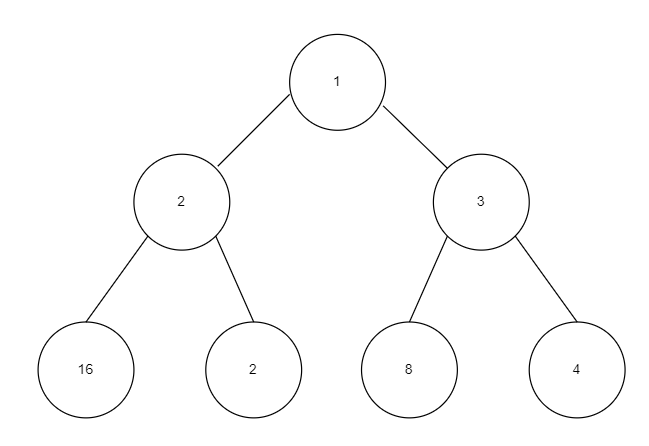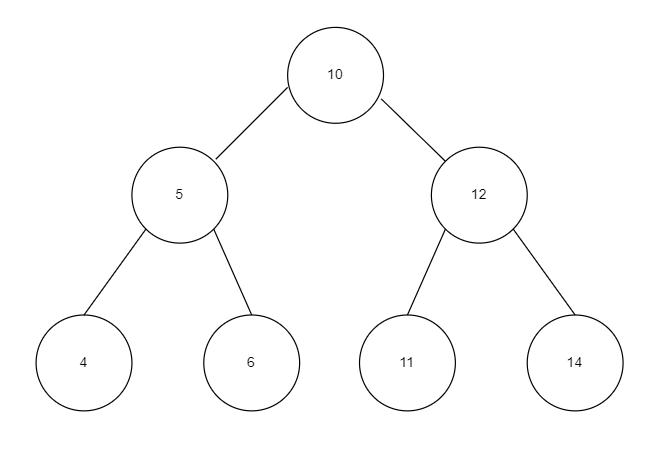# Iterative Approach to Check if a Binary Tree is BST or Not

## Introduction

Binary trees are an all-time favorite topic for coding interviews, and so is the Biinary search tree. Thus, it is important to master such topics. There are a large number of questions out there on binary trees and binary search trees, but if you get the hold of the topic right, you don’t need to practice them all, and you are good to go. Today we will be seeing one such frequently asked question in coding interviews, i.e., Check if a Binary Tree is BST or not. In this blog, we will be discussing the problem and its solutions from scratch.

## Understanding the Problem

The problem is fairly simple to understand. We are given a binary tree, and we have to check whether it’s a binary search tree or not and return ‘YES’ or ‘NO’, respectively.

A binary search tree is a binary tree in which the root of every subtree is greater than every node in its left subtree, and the root is less than every node in its right subtree.

You will get a better understanding by the following examples.This is not a BST as nodes in left subtree have values greater than root(1 < 2,1 < 16).This is a BST as the root of every subtree is greater than every node in its left subtree, and the root is less than every node in its right subtree.

## Intuition

The idea is to use something related to inorder traversal. As for a BST, its inorder traversal should give us a sorted array. Thus we will do something similar to it using a stack. Thus using a stack, we will perform the inorder traversal along the tree, but simultaneously, we will also maintain a ‘PREVIOUS’ pointer which will store the previously visited node during the inorder traversal and if at any point the current value of inorder traversal is less than the ‘PREVIOUS’ we will return false as then the binary tree cannot be BST. If we can perform a complete inorder traversal without violating the above condition, we will return true.

Things will become more clear from the following code.

## Code

``````#include <iostream>
#include <stack>
using namespace std;

// Structure of a tree node.
class BinaryTreeNode
{
public:
int data;
BinaryTreeNode *left;
BinaryTreeNode *right;

// Constructor.
BinaryTreeNode(int data)
{
this->data = data;
left = NULL;
right = NULL;
}
};

// This function will check if the given Binary Tree is BST or not.
bool checkTreeIsBST(BinaryTreeNode *root)
{
// Stack used for performing iterative inorder traversal.
stack<BinaryTreeNode*> s1;

// Stores previous visited node.
BinaryTreeNode *previous = NULL;

// Traverse the binary tree and perform Inorder traversal.
while (s1.size() != 0 || root != NULL)
{
// Traverse left subtree.
while (root != NULL)
{
// Insert root into ztack.
s1.push(root);
root = root->left;
}

root = s1.top();
s1.pop();

// If the value of root is less than 'PREVIOUS' value, then it cannot be BST. Thus we will return false.
if (previous != NULL)
{
if (root->data <= previous->data)
{
return false;
}
}

// Now root is our new 'PREVIOUS'
previous = root;

// Now right subtree will be traversed.
root = root->right;
}

return true;
}

// Driver Code
int main()
{
BinaryTreeNode *root = new BinaryTreeNode(10);
root->left = new BinaryTreeNode(5);
root->right = new BinaryTreeNode(12);
root->left->left = new BinaryTreeNode(4);
root->left->right = new BinaryTreeNode(6);
root->right->right = new BinaryTreeNode(14);
root->right->left = new BinaryTreeNode(11);

cout << checkTreeIsBST(root);
}``````

### Input### Output

``1``

### Time Complexity

O(N), where ‘N’ is the number of nodes in the tree.

As we are traversing every node in the tree only once.

### Space Complexity

O(N), where ‘N’ is the number of nodes in the tree.

As we are using a stack to store ‘N’ nodes to perform the inorder traversal.

## Key Takeaways

We saw how we could solve the problem ‘check if a binary tree is BST or not’ iteratively using the inorder traversal implemented by stack for iterative purposes. Now, Binary Trees and BST are wide topics and are equally important. Thus it requires a lot of practice, But don’t worry, we have a solution for it. Jump to our practice platform CodeStudio to practice top problems on every topic, read interview experiences, and many more. Till then, Happy Coding!